## Buildings.Controls.Continuous

Package with models for discrete time controls

### Information

Extends from Modelica.Icons.Package (Icon for standard packages).

### Package Content

Name DescriptionLimPID P, PI, PD, and PID controller with limited output, anti-windup compensation and setpoint weighting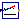NumberOfRequests Outputs the number of signals that are above/below a certain threshold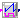OffTimer Records the time since the input changed to falsePIDHysteresis PID controller with anti-windup, output limiter and output hysteresisPIDHysteresisTimer PID controller with anti-windup, hysteresis and timer to prevent short cyclingSignalRanker Ranks output signals such that y[i] >= y[i+1]Examples Collection of models that illustrate model use and test modelsValidation Collection of validation models

##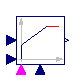Buildings.Controls.Continuous.LimPID

P, PI, PD, and PID controller with limited output, anti-windup compensation and setpoint weighting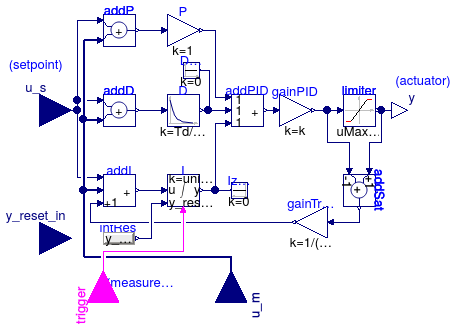### Information

This model is similar to Modelica.Blocks.Continuous.LimPID, except for the following changes:

1. It can be configured to have a reverse action.

If the parameter `reverseAction=false` (the default), then `u_m < u_s` increases the controller output, otherwise the controller output is decreased. Thus,

• for a heating coil with a two-way valve, set `reverseAction = false`,
• for a cooling coils with a two-way valve, set `reverseAction = true`.
2. It can be configured to enable an input port that allows resetting the controller output. The controller output can be reset as follows:

• If `reset = Buildings.Types.Reset.Disabled`, which is the default, then the controller output is never reset.
• If `reset = Buildings.Types.Reset.Parameter`, then a boolean input signal `trigger` is enabled. Whenever the value of this input changes from `false` to `true`, the controller output is reset by setting `y` to the value of the parameter `y_reset`.
• If `reset = Buildings.Types.Reset.Input`, then a boolean input signal `trigger` is enabled. Whenever the value of this input changes from `false` to `true`, the controller output is reset by setting `y` to the value of the input signal `y_reset_in`.

Note that this controller implements an integrator anti-windup. Therefore, for most applications, keeping the default setting of `reset = Buildings.Types.Reset.Disabled` is sufficient. Examples where it may be beneficial to reset the controller output are situations where the equipment control input should continuously increase as the equipment is switched on, such as as a light dimmer that may slowly increase the luminance, or a variable speed drive of a motor that should continuously increase the speed.

3. The parameter `limitsAtInit` has been removed.
4. Some parameters assignments in the instances have been made final.

Extends from Modelica.Blocks.Interfaces.SVcontrol (Single-Variable continuous controller).

### Parameters

TypeNameDefaultDescription
SimpleControllercontrollerTypeModelica.Blocks.Types.Simple...Type of controller
Realk1Gain of controller 
TimeTi0.5Time constant of Integrator block [s]
TimeTd0.1Time constant of Derivative block [s]
RealyMax1Upper limit of output
RealyMin0Lower limit of output
Realwp1Set-point weight for Proportional block (0..1)
Realwd0Set-point weight for Derivative block (0..1)
RealNi0.9Ni*Ti is time constant of anti-windup compensation
RealNd10The higher Nd, the more ideal the derivative block
BooleanreverseActionfalseSet to true for throttling the water flow rate through a cooling coil controller
Initialization
InitPIDinitTypeModelica.Blocks.Types.InitPI...Type of initialization (1: no init, 2: steady state, 3: initial state, 4: initial output)
Realxi_start0Initial or guess value value for integrator output (= integrator state)
Realxd_start0Initial or guess value for state of derivative block
Realy_start0Initial value of output
Integrator reset
ResetresetBuildings.Types.Reset.DisabledType of controller output reset
Realy_resetxi_startValue to which the controller output is reset if the boolean trigger has a rising edge, used if reset == Buildings.Types.Reset.Parameter
Booleanstricttrue= true, if strict limits with noEvent(..)

### Connectors

TypeNameDescription
input RealInputu_sConnector of setpoint input signal
input RealInputu_mConnector of measurement input signal
output RealOutputyConnector of actuator output signal
input BooleanInputtriggerResets the controller output when trigger becomes true
input RealInputy_reset_inInput signal for state to which integrator is reset, enabled if reset = Buildings.Types.Reset.Input

##Buildings.Controls.Continuous.NumberOfRequests

Outputs the number of signals that are above/below a certain threshold### Information

Block that outputs the number of inputs that exceed a threshold. The parameter `kind` is used to determine the kind of the inequality. The table below shows the allowed settings.

Value of parameter `kind` Output signal incremented by 1 for each i ∈ {1, ..., nin} if
0 `u[i] > threShold`
1 `u[i] ≥ threShold`
2 `u[i] ≤ threShold`
3 `u[i] < threShold`

This model may be used to check how many rooms exceed a temperature threshold.

Extends from Modelica.Blocks.Icons.Block (Basic graphical layout of input/output block).

### Parameters

TypeNameDefaultDescription
Integernin Number of inputs
RealthreShold0Threshold
Integerkind Set to 0 for u>threShold, to 1 for >=, to 2 for <= or to 3 for <

### Connectors

TypeNameDescription
output IntegerOutputyNumber of input signals that violate the threshold
input RealInputu[nin]Input signals

### Modelica definition

block NumberOfRequests "Outputs the number of signals that are above/below a certain threshold" extends Modelica.Blocks.Icons.Block; parameter Integer nin "Number of inputs"; parameter Real threShold=0 "Threshold"; parameter Integer kind "Set to 0 for u>threShold, to 1 for >=, to 2 for <= or to 3 for <"; Modelica.Blocks.Interfaces.IntegerOutput y "Number of input signals that violate the threshold"; Modelica.Blocks.Interfaces.RealInput u[nin] "Input signals"; algorithm y := 0; for i in 1:nin loop if kind == 0 then if u[i] > threShold then y := y + 1; end if; end if; if kind == 1 then if u[i] >= threShold then y := y + 1; end if; end if; if kind == 2 then if u[i] <= threShold then y := y + 1; end if; end if; if kind == 3 then if u[i] < threShold then y := y + 1; end if; end if; end for; end NumberOfRequests;

##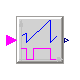Buildings.Controls.Continuous.OffTimer

Records the time since the input changed to false### Information

Timer that starts at the initial time with a value of 0, and gets reset each time the input signal switches to `false`.

For example, if the simulation starts at t = 10 and at t=11, the input becomes `false`, then the timer outputs y=t-10 for t < 11, and y=t-11 afterwards, unless the input becomes false again.

Extends from Modelica.Blocks.Interfaces.partialBooleanBlockIcon (This icon will be removed in future Modelica versions, use Modelica.Blocks.Icons.PartialBooleanBlock instead.).

### Connectors

TypeNameDescription
input BooleanInputuConnector of Boolean input signal
output RealOutputyConnector of Real output signal

### Modelica definition

model OffTimer "Records the time since the input changed to false" extends Modelica.Blocks.Interfaces.partialBooleanBlockIcon; Modelica.Blocks.Interfaces.BooleanInput u "Connector of Boolean input signal"; Modelica.Blocks.Interfaces.RealOutput y "Connector of Real output signal"; protected discrete Modelica.SIunits.Time entryTime "Time instant when u became true"; initial equation pre(entryTime) = time; equation when (not u) then entryTime = time; end when; y = time - entryTime; end OffTimer;

##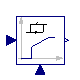Buildings.Controls.Continuous.PIDHysteresis

PID controller with anti-windup, output limiter and output hysteresis### Information

Block of a controller for set point tracking with a hysteresis element that switches the controller on and off.

If the controller is off, and the control error becomes larger than `eOn`, then the controller switches to on and remains on until the control error is smaller than `eOff`. When the controller is on, the set point tracking can be done using a P-, PI-, or PID-controller. In its off-mode, the control output is zero. Thus, the parameters `yMin` and `yMax` are used to constrain the output of the controller during its on mode only. This can be used, for example, to modulate a device between 0.3 and 1.0, and switch it to off when the control error is small enough.

Extends from Modelica.Blocks.Interfaces.SVcontrol (Single-Variable continuous controller).

### Parameters

TypeNameDefaultDescription
Hysteresis
RealeOn1if off and control error > eOn, switch to set point tracking
RealeOff-eOnif on and control error < eOff, set y=0
Booleanpre_y_startfalseValue of hysteresis output at initial time
Set point tracking
SimpleControllercontrollerTypeModelica.Blocks.Types.Simple...Type of controller
Realk1Gain of controller
TimeTi Time constant of Integrator block [s]
TimeTd Time constant of Derivative block [s]
RealyMax1Upper limit of output
RealyMin0Lower limit of output
Realwp1Set-point weight for Proportional block (0..1)
Realwd0Set-point weight for Derivative block (0..1)
RealNi0.9Ni*Ti is time constant of anti-windup compensation
RealNd10The higher Nd, the more ideal the derivative block
BooleanreverseActionfalseSet to true to enable reverse action (such as for a cooling coil controller)
Initialization
InitPIDinitTypeModelica.Blocks.Types.InitPI...Type of initialization (1: no init, 2: steady state, 3: initial state, 4: initial output)
Realxi_start0Initial or guess value value for integrator output (= integrator state)
Realxd_start0Initial or guess value for state of derivative block
Realy_start0Initial value of output
Booleanstricttrue= true, if strict limits with noEvent(..)

### Connectors

TypeNameDescription
input RealInputu_sConnector of setpoint input signal
input RealInputu_mConnector of measurement input signal
output RealOutputyConnector of actuator output signal

### Modelica definition

model PIDHysteresis "PID controller with anti-windup, output limiter and output hysteresis" extends Modelica.Blocks.Interfaces.SVcontrol; parameter Real eOn=1 "if off and control error > eOn, switch to set point tracking"; parameter Real eOff=-eOn "if on and control error < eOff, set y=0"; parameter Boolean pre_y_start=false "Value of hysteresis output at initial time"; parameter Modelica.Blocks.Types.SimpleController controllerType=Modelica.Blocks.Types.SimpleController.PID "Type of controller"; parameter Real k=1 "Gain of controller"; parameter Modelica.SIunits.Time Ti "Time constant of Integrator block"; parameter Modelica.SIunits.Time Td "Time constant of Derivative block"; parameter Real yMax=1 "Upper limit of output"; parameter Real yMin=0 "Lower limit of output"; parameter Real wp=1 "Set-point weight for Proportional block (0..1)"; parameter Real wd=0 "Set-point weight for Derivative block (0..1)"; parameter Real Ni=0.9 "Ni*Ti is time constant of anti-windup compensation"; parameter Real Nd=10 "The higher Nd, the more ideal the derivative block"; parameter Boolean reverseAction=false "Set to true to enable reverse action (such as for a cooling coil controller)"; parameter Modelica.Blocks.Types.InitPID initType=Modelica.Blocks.Types.InitPID.DoNotUse_InitialIntegratorState "Type of initialization (1: no init, 2: steady state, 3: initial state, 4: initial output)"; parameter Real xi_start=0 "Initial or guess value value for integrator output (= integrator state)"; parameter Real xd_start=0 "Initial or guess value for state of derivative block"; parameter Real y_start=0 "Initial value of output"; parameter Boolean strict=true "= true, if strict limits with noEvent(..)"; LimPID PID( final controllerType=controllerType, final k=k, final Ti=Ti, final yMax=yMax, final yMin=yMin, final wp=wp, final wd=wd, final Ni=Ni, final Nd=Nd, final initType=initType, final xi_start=xi_start, final xd_start=xd_start, final y_start=y_start, final Td=Td, final reverseAction=reverseAction, final strict=strict) "Controller for room temperature"; Modelica.Blocks.Logical.Hysteresis hys( final pre_y_start=pre_y_start, final uLow=eOff, final uHigh=eOn) "Hysteresis element to switch controller on and off"; Modelica.Blocks.Math.Feedback feeBac; protected Modelica.Blocks.Logical.Switch swi; Modelica.Blocks.Sources.Constant zer(final k=0) "Zero signal"; Modelica.Blocks.Logical.Switch swi1; equation assert(eOff < eOn, "Wrong controller parameters. Require eOff < eOn."); connect(zer.y, swi.u3); connect(swi.y, y); connect(u_m, PID.u_m); connect(hys.y, swi.u2); connect(PID.y, swi.u1); connect(u_s, feeBac.u1); connect(u_m, feeBac.u2); connect(feeBac.y, hys.u); connect(u_s, swi1.u1); connect(hys.y, swi1.u2); connect(u_m, swi1.u3); connect(swi1.y, PID.u_s); end PIDHysteresis;

##Buildings.Controls.Continuous.PIDHysteresisTimer

PID controller with anti-windup, hysteresis and timer to prevent short cycling### Information

Block of a controller for set point tracking with a hysteresis element that switches the controller on and off, and a timer that prevents the controller to short cycle.

The controller is similar to Buildings.Controls.Continuous.PIDHysteresis but in addition, it has a timer that prevents the controller from switching to on too fast. When the controller switches off, the timer starts and avoids the controller from switching on until `minOffTime` seconds elapsed.

Extends from Modelica.Blocks.Interfaces.SVcontrol (Single-Variable continuous controller).

### Parameters

TypeNameDefaultDescription
On/off controller
RealminOffTime600Minimum time that devices needs to be off before it can run again
RealeOn1if off and control error > eOn, switch to set point tracking
RealeOff-eOnif on and control error < eOff, set y=0
Booleanpre_y_startfalseValue of hysteresis output at initial time
Set point tracking
SimpleControllercontrollerTypeModelica.Blocks.Types.Simple...Type of controller
Realk1Gain of controller
TimeTi Time constant of Integrator block [s]
TimeTd Time constant of Derivative block [s]
RealyMax1Upper limit of modulating output
RealyMin0.3Lower limit of modulating output (before switch to 0)
Realwp1Set-point weight for Proportional block (0..1)
Realwd0Set-point weight for Derivative block (0..1)
RealNi0.9Ni*Ti is time constant of anti-windup compensation
RealNd10The higher Nd, the more ideal the derivative block
BooleanreverseActionfalseSet to true to enable reverse action (such as for a cooling coil controller)
Initialization
InitPIDinitTypeModelica.Blocks.Types.InitPI...Type of initialization (1: no init, 2: steady state, 3: initial state, 4: initial output)
Realxi_start0Initial or guess value value for integrator output (= integrator state)
Realxd_start0Initial or guess value for state of derivative block
Realy_start0Initial value of output
Booleanstricttrue= true, if strict limits with noEvent(..)

### Connectors

TypeNameDescription
input RealInputu_sConnector of setpoint input signal
input RealInputu_mConnector of measurement input signal
output RealOutputyConnector of actuator output signal
output RealOutputtOnTime since boiler switched on
output RealOutputtOffTime since boiler switched off
output BooleanOutputonOutputs true if boiler is on

### Modelica definition

model PIDHysteresisTimer "PID controller with anti-windup, hysteresis and timer to prevent short cycling" extends Modelica.Blocks.Interfaces.SVcontrol; parameter Real minOffTime=600 "Minimum time that devices needs to be off before it can run again"; parameter Real eOn=1 "if off and control error > eOn, switch to set point tracking"; parameter Real eOff=-eOn "if on and control error < eOff, set y=0"; parameter Boolean pre_y_start=false "Value of hysteresis output at initial time"; parameter Modelica.Blocks.Types.SimpleController controllerType=Modelica.Blocks.Types.SimpleController.PID "Type of controller"; parameter Real k=1 "Gain of controller"; parameter Modelica.SIunits.Time Ti "Time constant of Integrator block"; parameter Modelica.SIunits.Time Td "Time constant of Derivative block"; parameter Real yMax=1 "Upper limit of modulating output"; parameter Real yMin=0.3 "Lower limit of modulating output (before switch to 0)"; parameter Real wp=1 "Set-point weight for Proportional block (0..1)"; parameter Real wd=0 "Set-point weight for Derivative block (0..1)"; parameter Real Ni=0.9 "Ni*Ti is time constant of anti-windup compensation"; parameter Real Nd=10 "The higher Nd, the more ideal the derivative block"; parameter Boolean reverseAction=false "Set to true to enable reverse action (such as for a cooling coil controller)"; parameter Modelica.Blocks.Types.InitPID initType=Modelica.Blocks.Types.InitPID.DoNotUse_InitialIntegratorState "Type of initialization (1: no init, 2: steady state, 3: initial state, 4: initial output)"; parameter Real xi_start=0 "Initial or guess value value for integrator output (= integrator state)"; parameter Real xd_start=0 "Initial or guess value for state of derivative block"; parameter Real y_start=0 "Initial value of output"; parameter Boolean strict=true "= true, if strict limits with noEvent(..)"; Modelica.Blocks.Interfaces.RealOutput tOn "Time since boiler switched on"; Modelica.Blocks.Interfaces.RealOutput tOff "Time since boiler switched off"; LimPID con( final controllerType=controllerType, final k=k, final Ti=Ti, final Td=Td, final wp=wp, final wd=wd, final Ni=Ni, final Nd=Nd, final initType=initType, final xi_start=xi_start, final xd_start=xd_start, final y_start=y_start, final yMin=yMin, final yMax=yMax, final reverseAction=reverseAction, final strict=strict) "Controller to track setpoint"; OffTimer offHys; Modelica.Blocks.Logical.Timer onTimer; Modelica.Blocks.Logical.Timer offTimer; Modelica.Blocks.Interfaces.BooleanOutput on "Outputs true if boiler is on"; Modelica.Blocks.Math.Feedback feeBac; Modelica.Blocks.Logical.Hysteresis hys( final pre_y_start=pre_y_start, final uLow=eOff, final uHigh=eOn) "Hysteresis element to switch controller on and off"; protected Modelica.Blocks.Sources.Constant zer(final k=0) "Zero signal"; Modelica.Blocks.Logical.Switch switch2; Modelica.Blocks.Logical.GreaterEqualThreshold greaterEqualThreshold(threshold =minOffTime); Modelica.Blocks.Logical.And and3; Modelica.Blocks.Logical.Not not1; Modelica.Blocks.Logical.Switch switch1; equation connect(u_m, con.u_m); connect(zer.y, switch2.u3); connect(switch2.y, y); connect(and3.y, switch2.u2); connect(greaterEqualThreshold.y, and3.u1); connect(offHys.y, greaterEqualThreshold.u); connect(con.y, switch2.u1); connect(onTimer.y, tOn); connect(offTimer.y, tOff); connect(and3.y, on); connect(and3.y, onTimer.u); connect(and3.y, not1.u); connect(not1.y, offTimer.u); connect(and3.y, switch1.u2); connect(u_s, switch1.u1); connect(u_m, switch1.u3); connect(switch1.y, con.u_s); connect(u_s, feeBac.u1); connect(u_m, feeBac.u2); connect(feeBac.y, hys.u); connect(hys.y, offHys.u); connect(hys.y, and3.u2); end PIDHysteresisTimer;

##Buildings.Controls.Continuous.SignalRanker

Ranks output signals such that y[i] >= y[i+1]### Information

Block that sorts the input signal `u[:]` such that the output signal satisfies `y[i] >= y[i+1]` for all `i=1, ..., nin-1`.

This block may for example be used in a variable air volume flow controller to access the position of the dampers that are most open.

Extends from Modelica.Blocks.Interfaces.MIMO (Multiple Input Multiple Output continuous control block).

### Parameters

TypeNameDefaultDescription
Integernin1Number of inputs
IntegernoutninNumber of outputs

### Connectors

TypeNameDescription
input RealInputu[nin]Connector of Real input signals
output RealOutputy[nout]Connector of Real output signals

### Modelica definition

block SignalRanker "Ranks output signals such that y[i] >= y[i+1]" extends Modelica.Blocks.Interfaces.MIMO(final nout=nin); protected Real t "Temporary variable"; algorithm y[:] := u[:]; for i in 1:nin loop for j in 1:nin - 1 loop if y[j] < y[j + 1] then t := y[j + 1]; y[j + 1] := y[j]; y[j] := t; end if; end for; end for; end SignalRanker;